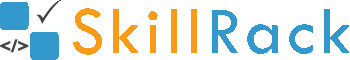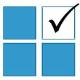ProgramID- 11189SkillRack
Right Angled Triangle or Not

The program is to accept three sides of a triangle and check if the triangle is a right angled triangle or not.
Note: In a right angled triangle the square of the hypotenuse is equal to the sum of the squares of the other two sides.

Boundary Condition(s):
1 <= Length of the sides <= 1000

Input Format:
The first line contains three integers denoting the length of the sides separated by a space.

Output Format:
The first line contains yes or no.

Example Input/Output 1:
Input:
5 4 3

Output:
yes

Example Input/Output 2:
Input:
8 5 6

Output:
no

Max Execution Time Limit: 500 millisecs